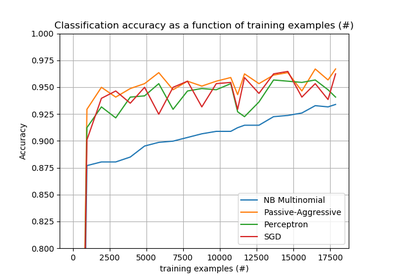# `sklearn.naive_bayes`.MultinomialNB¶

class `sklearn.naive_bayes.``MultinomialNB`(alpha=1.0, fit_prior=True, class_prior=None)[source]

Naive Bayes classifier for multinomial models

The multinomial Naive Bayes classifier is suitable for classification with discrete features (e.g., word counts for text classification). The multinomial distribution normally requires integer feature counts. However, in practice, fractional counts such as tf-idf may also work.

Read more in the User Guide.

Parameters: alpha : float, optional (default=1.0) Additive (Laplace/Lidstone) smoothing parameter (0 for no smoothing). fit_prior : boolean, optional (default=True) Whether to learn class prior probabilities or not. If false, a uniform prior will be used. class_prior : array-like, size (n_classes,), optional (default=None) Prior probabilities of the classes. If specified the priors are not adjusted according to the data. class_log_prior_ : array, shape (n_classes, ) Smoothed empirical log probability for each class. intercept_ : property Mirrors `class_log_prior_` for interpreting MultinomialNB as a linear model. feature_log_prob_ : array, shape (n_classes, n_features) Empirical log probability of features given a class, `P(x_i|y)`. coef_ : property Mirrors `feature_log_prob_` for interpreting MultinomialNB as a linear model. class_count_ : array, shape (n_classes,) Number of samples encountered for each class during fitting. This value is weighted by the sample weight when provided. feature_count_ : array, shape (n_classes, n_features) Number of samples encountered for each (class, feature) during fitting. This value is weighted by the sample weight when provided.

Notes

For the rationale behind the names coef_ and intercept_, i.e. naive Bayes as a linear classifier, see J. Rennie et al. (2003), Tackling the poor assumptions of naive Bayes text classifiers, ICML.

References

C.D. Manning, P. Raghavan and H. Schuetze (2008). Introduction to Information Retrieval. Cambridge University Press, pp. 234-265. http://nlp.stanford.edu/IR-book/html/htmledition/naive-bayes-text-classification-1.html

Examples

```>>> import numpy as np
>>> X = np.random.randint(5, size=(6, 100))
>>> y = np.array([1, 2, 3, 4, 5, 6])
>>> from sklearn.naive_bayes import MultinomialNB
>>> clf = MultinomialNB()
>>> clf.fit(X, y)
MultinomialNB(alpha=1.0, class_prior=None, fit_prior=True)
>>> print(clf.predict(X[2:3]))

```

Methods

 `fit`(X, y[, sample_weight]) Fit Naive Bayes classifier according to X, y `get_params`([deep]) Get parameters for this estimator. `partial_fit`(X, y[, classes, sample_weight]) Incremental fit on a batch of samples. `predict`(X) Perform classification on an array of test vectors X. `predict_log_proba`(X) Return log-probability estimates for the test vector X. `predict_proba`(X) Return probability estimates for the test vector X. `score`(X, y[, sample_weight]) Returns the mean accuracy on the given test data and labels. `set_params`(**params) Set the parameters of this estimator.
`__init__`(alpha=1.0, fit_prior=True, class_prior=None)[source]
`fit`(X, y, sample_weight=None)[source]

Fit Naive Bayes classifier according to X, y

Parameters: X : {array-like, sparse matrix}, shape = [n_samples, n_features] Training vectors, where n_samples is the number of samples and n_features is the number of features. y : array-like, shape = [n_samples] Target values. sample_weight : array-like, shape = [n_samples], (default=None) Weights applied to individual samples (1. for unweighted). self : object Returns self.
`get_params`(deep=True)[source]

Get parameters for this estimator.

Parameters: deep : boolean, optional If True, will return the parameters for this estimator and contained subobjects that are estimators. params : mapping of string to any Parameter names mapped to their values.
`partial_fit`(X, y, classes=None, sample_weight=None)[source]

Incremental fit on a batch of samples.

This method is expected to be called several times consecutively on different chunks of a dataset so as to implement out-of-core or online learning.

This is especially useful when the whole dataset is too big to fit in memory at once.

This method has some performance overhead hence it is better to call partial_fit on chunks of data that are as large as possible (as long as fitting in the memory budget) to hide the overhead.

Parameters: X : {array-like, sparse matrix}, shape = [n_samples, n_features] Training vectors, where n_samples is the number of samples and n_features is the number of features. y : array-like, shape = [n_samples] Target values. classes : array-like, shape = [n_classes] (default=None) List of all the classes that can possibly appear in the y vector. Must be provided at the first call to partial_fit, can be omitted in subsequent calls. sample_weight : array-like, shape = [n_samples] (default=None) Weights applied to individual samples (1. for unweighted). self : object Returns self.
`predict`(X)[source]

Perform classification on an array of test vectors X.

Parameters: X : array-like, shape = [n_samples, n_features] C : array, shape = [n_samples] Predicted target values for X
`predict_log_proba`(X)[source]

Return log-probability estimates for the test vector X.

Parameters: X : array-like, shape = [n_samples, n_features] C : array-like, shape = [n_samples, n_classes] Returns the log-probability of the samples for each class in the model. The columns correspond to the classes in sorted order, as they appear in the attribute classes_.
`predict_proba`(X)[source]

Return probability estimates for the test vector X.

Parameters: X : array-like, shape = [n_samples, n_features] C : array-like, shape = [n_samples, n_classes] Returns the probability of the samples for each class in the model. The columns correspond to the classes in sorted order, as they appear in the attribute classes_.
`score`(X, y, sample_weight=None)[source]

Returns the mean accuracy on the given test data and labels.

In multi-label classification, this is the subset accuracy which is a harsh metric since you require for each sample that each label set be correctly predicted.

Parameters: X : array-like, shape = (n_samples, n_features) Test samples. y : array-like, shape = (n_samples) or (n_samples, n_outputs) True labels for X. sample_weight : array-like, shape = [n_samples], optional Sample weights. score : float Mean accuracy of self.predict(X) wrt. y.
`set_params`(**params)[source]

Set the parameters of this estimator.

The method works on simple estimators as well as on nested objects (such as pipelines). The latter have parameters of the form `<component>__<parameter>` so that it’s possible to update each component of a nested object.

Returns: self :

## Examples using `sklearn.naive_bayes.MultinomialNB`¶Out-of-core classification of text documentsClassification of text documents using sparse features